# 第四章 数据抓取与机器学习算法¶

## 4.2 爬虫示例¶

### 新浪微博¶

https://github.com/followyourheart/sina-weibo-crawler

## 4.3 numpy 快查¶

import numpy as np
a = np.arange(1,5)
data_type = [('name','S10'), ('height', 'float'), ('age', int)]
values = [('Arthur', 1.8, 41), ('Lancelot', 1.9, 38),
b = np.array(values, dtype=data_type)
c = np.arange(6,10)

# 符号
np.sign(a)

# 数组最大值
a.max()

# 数组最小值
a.max()

# 区间峰峰值
a.ptp()

# 乘积
a.prod()

# 累积
a.cumprod()

# 平均值
a.mean()

# 中值
a.median()

# 差分
np.diff(a)

# 方差
np.var(a)

# 元素条件查找，返回index的array
np.where(a>2)

# 返回第2，3，5个元素的array
np.take(a, np.array(1,2,4))

# 排序
np.msort(a)
np.sort(b, kind='mergesort', order='height')

# 均分，奇数个元素的array不可分割为偶数。
np.split(b,2)

# 创建单位矩阵
np.eye(3)

# 最小二乘，参数为[x,y,degree]，degree为多项式的最高次幂，返回值为所有次幂的系数
np.polyfit(a,c,1)


## 4.4 监督学习常用算法及Python实现¶

### 信息分类基础¶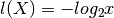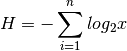def chooseBestFeatureToSplit(dataSet):
numFeatures = len(dataSet) - 1      #the last column is used for the labels
baseEntropy = calcShannonEnt(dataSet)
bestInfoGain = 0.0; bestFeature = -1
for i in range(numFeatures):        #iterate over all the features
featList = [example[i] for example in dataSet]#create a list of all the examples of this feature
uniqueVals = set(featList)       #get a set of unique values
newEntropy = 0.0
for value in uniqueVals:
subDataSet = splitDataSet(dataSet, i, value)
prob = len(subDataSet)/float(len(dataSet))
newEntropy += prob * calcShannonEnt(subDataSet)
infoGain = baseEntropy - newEntropy     #calculate the info gain; ie reduction in entropy
if (infoGain > bestInfoGain):       #compare this to the best gain so far
bestInfoGain = infoGain         #if better than current best, set to best
bestFeature = i
return bestFeature                      #returns an integer



### K邻近算法¶

kNN的算法模型如下：

• 计算已知类别数据集中的点与当前点之间的距离
• 按照距离递增依次排序
• 选取与当前点距离最小的k个点
• 确定前k个点所在类别的出现频率
• 返回前k个点出现频率最高的类别作为当前点的预测分类

def classify0(inX, dataSet, labels, k):
dataSetSize = dataSet.shape
diffMat = tile(inX, (dataSetSize,1)) - dataSet
sqDiffMat = diffMat**2
sqDistances = sqDiffMat.sum(axis=1)
distances = sqDistances**0.5
sortedDistIndicies = distances.argsort()
classCount={}
for i in range(k):
voteIlabel = labels[sortedDistIndicies[i]]
classCount[voteIlabel] = classCount.get(voteIlabel,0) + 1
sortedClassCount = sorted(classCount.iteritems(), key=operator.itemgetter(1), reverse=True)
return sortedClassCount



### 决策树¶

 def createTree(dataSet,labels):
classList = [example[-1] for example in dataSet]
if classList.count(classList) == len(classList):
return classList     #stop splitting when all of the classes are equal
if len(dataSet) == 1:    #stop splitting when there are no more features in dataSet
return majorityCnt(classList)
bestFeat = chooseBestFeatureToSplit(dataSet)
bestFeatLabel = labels[bestFeat]
myTree = {bestFeatLabel:{}}
del(labels[bestFeat])
featValues = [example[bestFeat] for example in dataSet]
uniqueVals = set(featValues)
for value in uniqueVals:
subLabels = labels[:]       #copy all of labels, so trees don't mess up existing labels
myTree[bestFeatLabel][value] = createTree(splitDataSet(dataSet, bestFeat, value),subLabels)
return myTree

找到影响最大的特征bestFeat后，再创建此特征下的分类向量创建子树向量，然后将bestFeat分离后继续迭代，直至所有特征都转换成决策节点。

原始数据比如：

no-surfacing flippers  fish
1       yes         yes     yes
2       yes         yes     yes
3       yes         no      no
4       no          yes     no
5       no          yes     no

会生成如下决策树：

no-surfacing?
/    \
no/      \yes
fish(no)  flippers?
/ \
no/   \yes
fish(no)  fish(yes)

表示成JSON格式，即python字典：

{'no surfacing':{0:'no',1:{'flippers':{0:'no',1:'yes'}}}

构建决策树的方法比较多，也可使用C4.5和CART算法。


def classify(inputTree,featLabels,testVec):
firstStr = inputTree.keys()
secondDict = inputTree[firstStr]
featIndex = featLabels.index(firstStr)
key = testVec[featIndex]
valueOfFeat = secondDict[key]
if isinstance(valueOfFeat, dict):
classLabel = classify(valueOfFeat, featLabels, testVec)
else: classLabel = valueOfFeat
return classLabel



 def storeTree(inputTree,filename):
import pickle
fw = open(filename,'w')
pickle.dump(inputTree,fw)
fw.close()



### 朴素贝叶斯¶

总共7个石子在A、B两个桶中，A桶中有2黑2白，B桶中有2黑1白。已知条件为石子来自B桶，那么它是白色石子的概率可表示为：

P(white|B)=P(B|white)P(white)/P(B)

P(B|A)=P(A|B)P(B)/P(A)



def trainNB0(trainMatrix,trainCategory):
numTrainDocs = len(trainMatrix)
numWords = len(trainMatrix)
p0Num = ones(numWords); p1Num = ones(numWords)      #change to ones()
p0Denom = 2.0; p1Denom = 2.0                        #change to 2.0
for i in range(numTrainDocs):
if trainCategory[i] == 1:
p1Num += trainMatrix[i]
p1Denom += sum(trainMatrix[i])
else:
p0Num += trainMatrix[i]
p0Denom += sum(trainMatrix[i])
p1Vect = log(p1Num/p1Denom)          #change to log()
p0Vect = log(p0Num/p0Denom)          #change to log()



def classifyNB(vec2Classify, p0Vec, p1Vec, pClass1):
p1 = sum(vec2Classify * p1Vec) + log(pClass1)    #element-wise mult
p0 = sum(vec2Classify * p0Vec) + log(1.0 - pClass1)
if p1 > p0:
return 1
else:
return 0

vec2Classify即为要分类的向量，形如trainMatrix，随后的三个参数为trainNB0所返回。p1、p0可以理解为期望概率值，比较两者大小即可划分。


def testingNB():
myVocabList = createVocabList(listOPosts)
trainMat=[]
for postinDoc in listOPosts:
trainMat.append(setOfWords2Vec(myVocabList, postinDoc))
p0V,p1V,pAb = trainNB0(array(trainMat),array(listClasses))
testEntry = ['love', 'my', 'dalmation']
thisDoc = array(setOfWords2Vec(myVocabList, testEntry))
print testEntry,'classified as: ',classifyNB(thisDoc,p0V,p1V,pAb)
testEntry = ['stupid', 'garbage']
thisDoc = array(setOfWords2Vec(myVocabList, testEntry))
print testEntry,'classified as: ',classifyNB(thisDoc,p0V,p1V,pAb)


### Logistic回归¶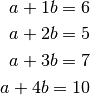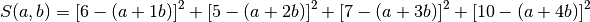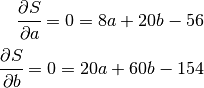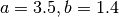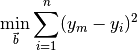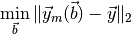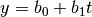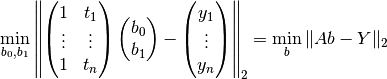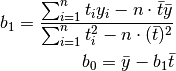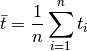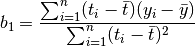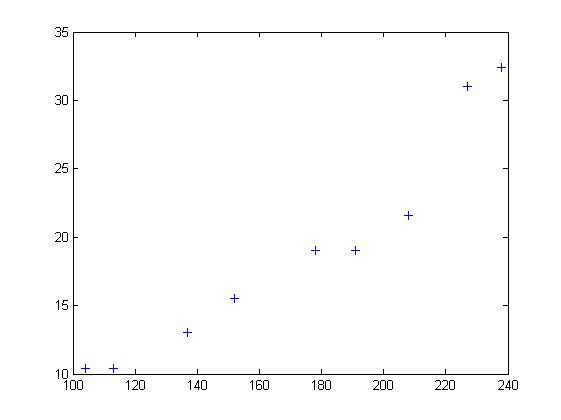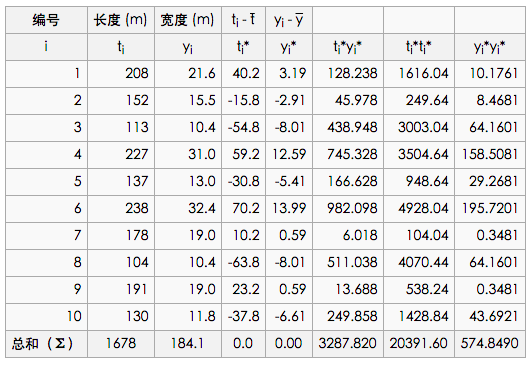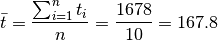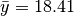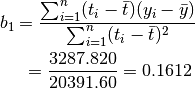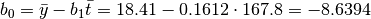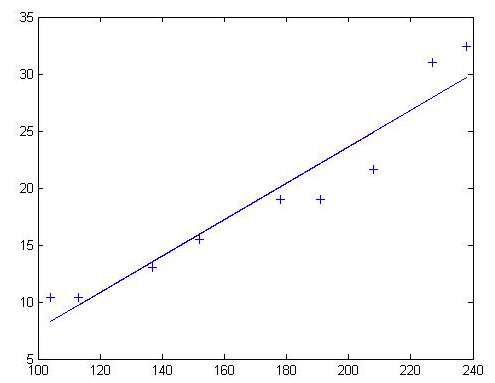Sigmoid函数

Sigmoid函数具有单位阶跃函数的性质，公式表示为：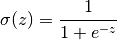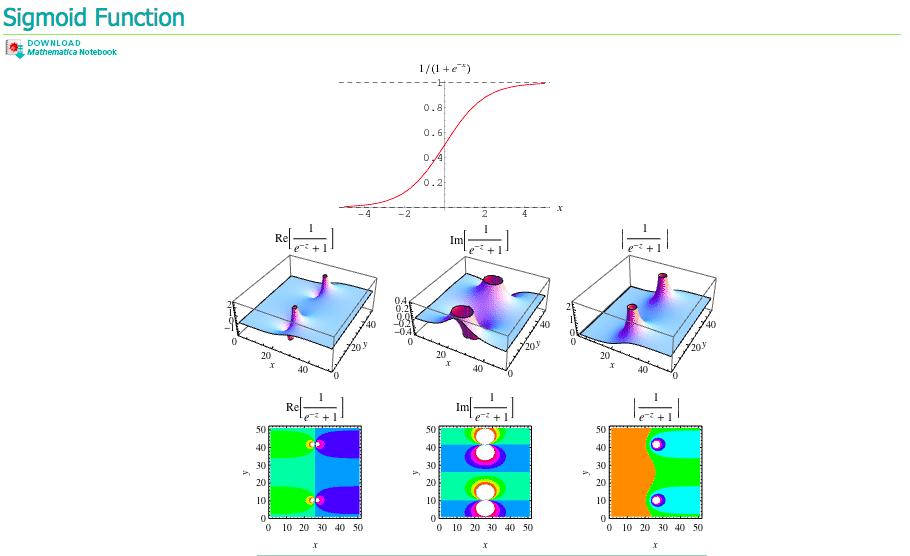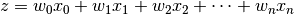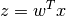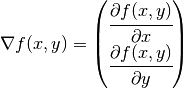def gradAscent(dataMatIn, classLabels):
dataMatrix = mat(dataMatIn)             #convert to NumPy matrix
labelMat = mat(classLabels).transpose() #convert to NumPy matrix
m,n = shape(dataMatrix)
alpha = 0.001
maxCycles = 500
weights = ones((n,1))
for k in range(maxCycles):              #heavy on matrix operations
h = sigmoid(dataMatrix*weights)     #matrix mult
error = (labelMat - h)              #vector subtraction
weights = weights + alpha * dataMatrix.transpose()* error #matrix mult
return weights



### SVM¶

SVM（Supprot Vector Machines）即支持向量机，完全理解其理论知识对数学要求较高。

## 4.6 数据可视化¶

### 数据统计¶

Gephi

GraphViz

python-matplotlib

Microsoft Excel 2013 PowerView

### 地理位置表示¶

MaxMind GeoIP

Microsoft Excel 2013 PowerView使用示例

Weka

Netlogo

SciKit

Pandas